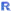# Standard R

R analyses that are "built-in" to Q are referred to as Standard R. These are analyses where the R code has been connected to a graphical user interface (e.g., is available from a menu, options can be accessed from checkboxes, etc.). Standard R thus consists of:

• Pre-written R code. The code has been written in a way that makes it relatively easy to use, so that similar things are done in similar ways and standard terminology is used where practical. See Standard R Style Guide.
• A graphical user interface (GUI), so that users can select the inputs for their analyses using menus and controls on a form (as opposed to writing code). See R GUI Controls).

There are a variety of ways to create Standard R analyses:

• Creating an R Output, and adding controls into Properties > INPUTS JAVASCRIPT in the Object Inspector.
• Creating a QScript which adds an R Output or R Variable.
• Use the Create or Automate menus in Q, choosing options with an R logo (i.e.,). This automatically creates an R Output or R Variable. There are two different ways that this works at an underlying level:
1. Standard R Pages. These are pages on the wiki, which match options in the menus. For example, if you select Create > Regression > Linear Regression, Q:
• Inserts an R Output.
• Goes to the Regression - Linear Regression page on this wiki, extracts the code in the first of the code boxes, and pastes it into the R Output's INPUTS JAVASCRIPT box.
• Extracts the code from the second box and pastes it into the R Code box.
• Un-checks the Automatic button (above the R Code box), so that the user can manually modify the controls without the code automatically running.
2. Using QScript (see the discussion above).
• Using Search and choosing options with an R logo. This works in the same way as when using a menu.